* You are viewing the archive for the ‘Formula-27 on Space’ Category

## Formula-27 on Space

Polyhedra of the Platonic Solids: From Meta-cube to the Tetraktys. The following diagrams illustrate the polyhedron sequences embedded inside the Tetraktys generated by the Meta-Cube for the propagation of the Involution Logic-Information and the Evolution of Information-Energy.

FORMATION OF THE TETRAKTYS STARTING WITH THE INTER-LOCKIG STAR-TETRAHEDRONUNFOLDING THE STAR-TETRAHEDRON

NOTICE THE CROSSPOLYHEDRONS WITH META-CUBE IN MIDDLE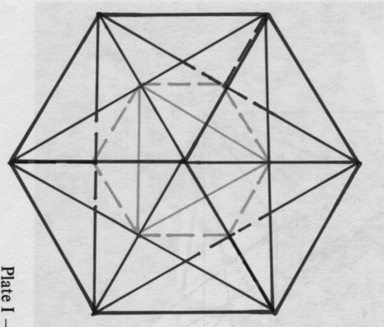FROM META-CUBE TO POLYHEDRONNOTICE THE CUBE IN THE MIDDLE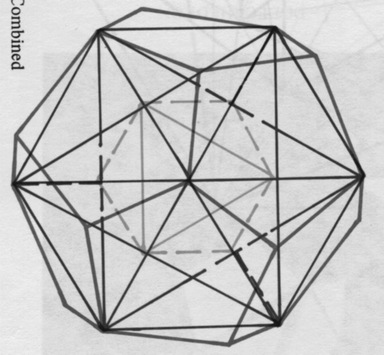AGAIN NOTICE THE CUBE IN THE MIDDLE

INVOLUTION—EVOLUTION THROUGH POLYHEDRON AND THE TETRAKTYS

TWO LEVELS OF THE ATOM:

(1) ARRANGING THE QUAKS AND LEPTONS AND FORCE LOGIC IN A STAR-TETRAHEDRON

STAGE (1): THE FORMATION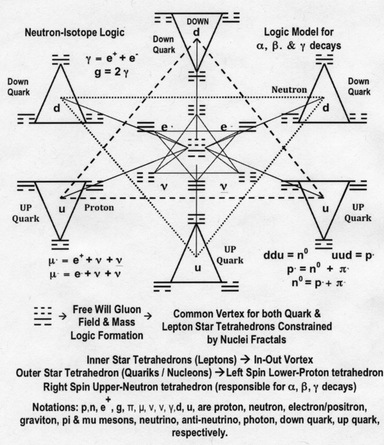STAGE (2): ENCLOSED IN SUCCESSIVE POLYHEDRONS OF THE NUCLEI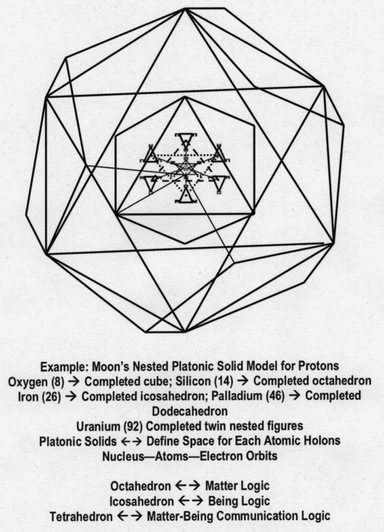Robert James Moon (14 February 1911 – 1 November 1989)

“He pioneered work on the fundamental structure of the atomic nucleus based on platonic solids. In the 1984 – 1986 period, Moon came up with his proposal for a geometric ordering of protons and neutrons in the atomic nucleus based on nested platonic solids. This “Moon Model”, was inspired by Johannes Kepler’s conception of the solar system, as described in Kepler’s work Mysterium Cosmographicum. The model is also inspired by the discovery by von Klitzing of the quantum Hall effect, leading Moon to believe that space itself is quantized, and that the nucleons are positioned at discrete locations, i.e. at the vertices of a set of nested platonic solids. An example is show below…” Laurence Hecht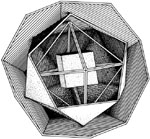AGAIN NOTICE THE CUBE (THE META-CUBE)

THE MOON MODEL OF THE NUCLEUS A nesting of four of the five Platonic solids , starting with the cube, then octahedron, then icosahedron, inside a dodecahedron complete the first shell. The 46vertices of the nested figure represent figure represent palladium, atomic, atomic number 46. To go beyond palladiun, an identical dodechedron is joined to the first one at a face…

What we have here are sets of quantized space unique for each nucleus following the principle of Platonic solids generated from the 3-D Star Tetrahedron or the Meta-Cube. Thus Formula-27 is at play. In other words, Space is constrained by the Polyhefra. Furthermore, the nuclei are constrained inside these Polyhedra. Thus, Space in this definition is quantized and it is only connected to time in the Holon of ict —-> NOW.

Meta-Cube / Phi Inside Formula-27 Mirror and inside the 172 Table

27-ISOTOPE LOGIC-INFORMATION SIDE OF THE MIRROR

27 Unseen Truth —–> 64 TRUTH

Element 87-172 in Phi Proportion, Upper Meta-Cube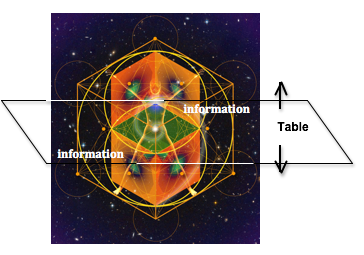Element 1-86 in Phi Proportion, Lower Meta-Cube

37 Visible Truth —–> 64 TRUTH

27-ISOPOTE INFORMATION-ENERGY SIDE OF THE MIRROR

Remember, the Radionics on the 172 Element Table, showing a + equal and opposite responds above naval for Elements 1 to 86 as compared to the below the naval responds for Elements 87 to 172. From the Information Mirror as shown above, the Upper Meta-Cube occupies the Logic-Elements with the corresponding Energy-Elements on the Bottom Meta-Cube. They both generated the opposite connecting Phi proportions, as show.

Upper and Lower Phi Proportion in the Human Body matches the Logic Phi proportion with the Energy Phi proportion in the radionics experiment as stated above, see illustration below.

Human Body in Uper-Lower Phi Proportion separated at the Navel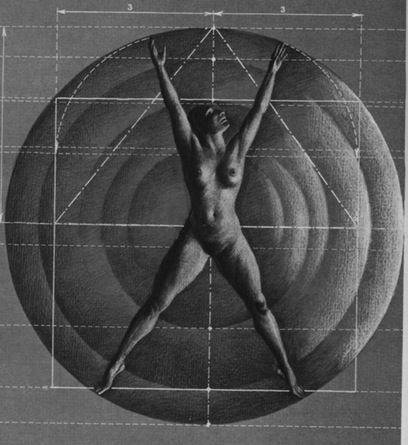Through the Information Mirror

• Phi is the Binding Information between Pre-Heaven—-Unseen Truth—-Pre-Quantum and Post-Heaven—–Visible Truth—-Quantum
• Phi is the Binding Information for Base-9 and Base-10
• Phi is the generator for all the Logic-Information-Energy Constants in Quantum Physics see Physical Constants Generated by φ (Phi)
• Phi is the connection between Living and Non-Living, i.e., between Being and Being-In-Matter
• Phi non-locally Teleports Space (Local) to NOW (Non-Local)
• ETC

These definitely demonstrates the “Powers” of this Information Mirror between Logic and Energy. These are the hidden inside the Meta-Cube for the discovery and for the reverse engineering of God’s Involution Logic. To understand further, lets again illustrates below the Mirror hidden in the Tetraktys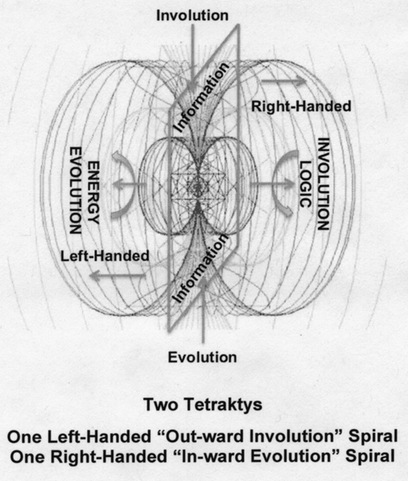The two Tetraktys are an Upper Logic Meta-Cube and a Lower Energy Meta-Cube. Thus, we have just derived the FORMULA-27 for the Imaginary Mirror of the Holon Space—-i—-Now.

FINALLY: Space must be Reinterpreted

Like the concepts of Mind—Soul—Spirit replaced the importance of Elementary Particles-forces, Space must be re-interpreted (or its interpretation must be expanded) as Information Coordinates together with others as expressed in the diagrams below.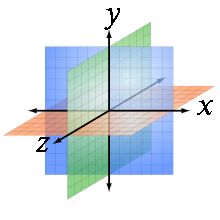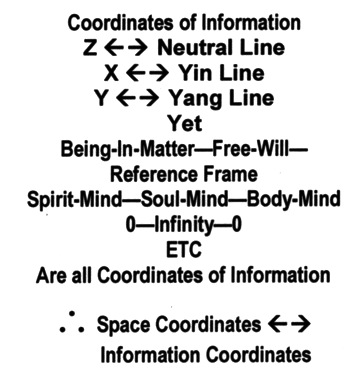AND THE FOLLOWING DIAGRAMS FOR ATOMS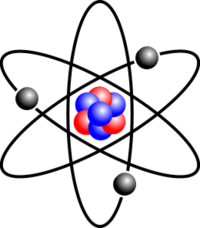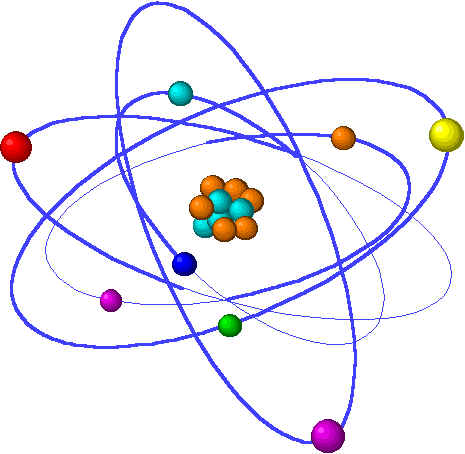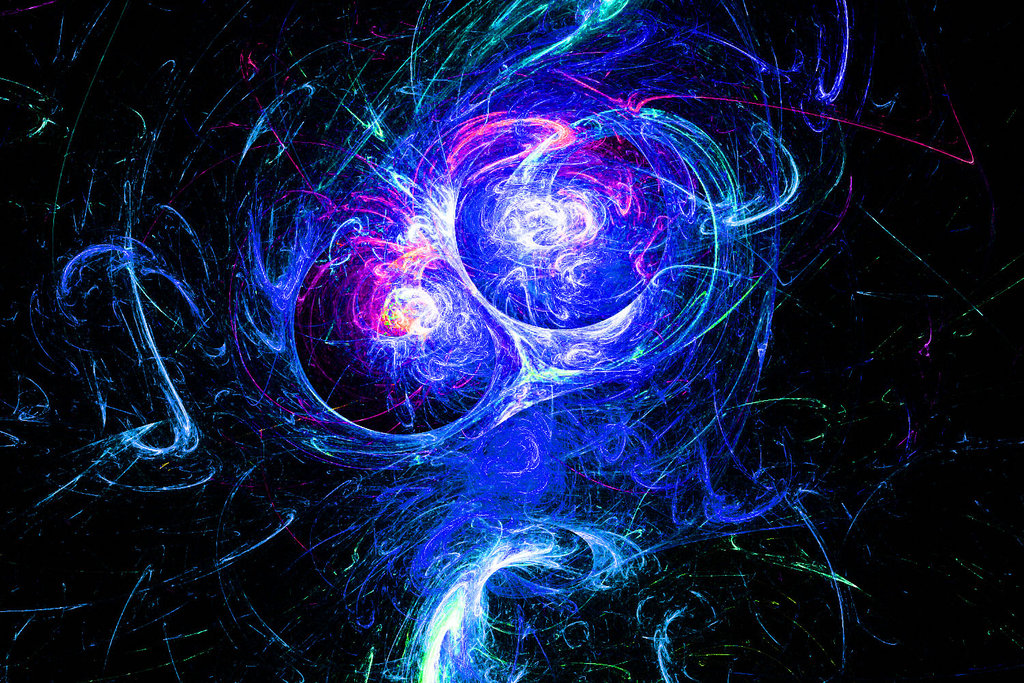COLLIDING ATOMS

From the Meta-Cube, Space is Unseen but the Coordinates of Information are Visible.

Finally, the HOLONS in FORMULA-27 become not only a TOE in Physics; but also a THEORY OF EVERYTHING FOR the Ancient’s Pre-Quantum Philosophy, Modern Day’s Quantum-Relativistic Physics, and the Future Bio Quantum in Human Science.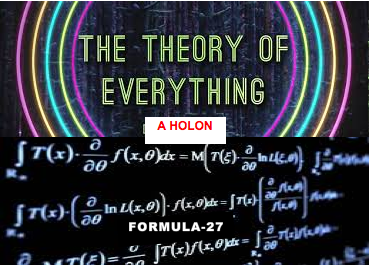Before we conclude, there is one last issue needs to consider from the Mimd-Being points of view. This is the concepts of Supersymmetry (Symmetry that connects Zero and Infinity, between the two sides of the Visible and Invisible Logic Mirror). The Paradigm’s view on this is illustrated below in the Posting.